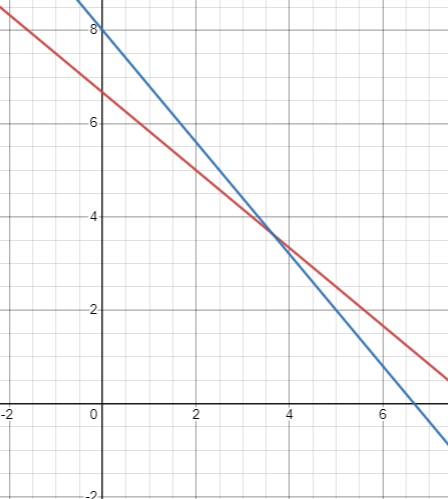Request a call back

A= {(x, y): x, y∈ I, X≥0, y≥0 and 5x + 6y ≤40} B= {(x, y): x, y∈ l, x≥0, y≥0 and 6x + 5y ≤ 40} Where I denotes set of integers, then n(A∩B) is
Asked by mohvijayjain12 | 22 Jun, 2022, 11:07: AMExpert Answer
A= {(x, y): x, y∈ I, X≥0, y≥0 and 5x + 6y ≤40} \
B= {(x, y): x, y∈ l, x≥0, y≥0 and 6x + 5y ≤ 40}
The graph isTherefore, x and y can be equal to or less than 6
When x = 0, there are 7 points
When x = 1, there are 6 points
When x = 2, there are 6 points
When x = 3, there are 5 points
When x = 4, there are 4 points
When x = 5, there are 3 points
When x = 6, there is only one point
Thus, n(A∩B) = 7 + 6 + 6 + 5 + 4 + 3 + 1 = 32

Answered by Renu Varma | 25 Jun, 2022, 11:47: AM
CBSE 11-science - Maths
Asked by mohvijayjain12 | 22 Jun, 2022, 11:07: AMANSWERED BY EXPERT
CBSE 11-science - Maths
Asked by swajaydixit04 | 19 Jun, 2020, 10:22: PMANSWERED BY EXPERT
CBSE 11-science - Maths
Asked by Topperlearning User | 30 Apr, 2014, 11:19: AMANSWERED BY EXPERT
CBSE 11-science - Maths
Asked by Topperlearning User | 30 Apr, 2014, 11:22: AMANSWERED BY EXPERT
CBSE 11-science - Maths
Asked by Topperlearning User | 04 Jun, 2014, 01:23: PMANSWERED BY EXPERT
CBSE 11-science - Maths
Asked by Topperlearning User | 04 Jun, 2014, 01:23: PMANSWERED BY EXPERT
CBSE 11-science - Maths
Asked by Topperlearning User | 04 Jun, 2014, 01:23: PMANSWERED BY EXPERT
CBSE 11-science - Maths
Asked by Topperlearning User | 04 Jun, 2014, 01:23: PMANSWERED BY EXPERT
CBSE 11-science - Maths
Asked by Topperlearning User | 04 Jun, 2014, 01:23: PMANSWERED BY EXPERT